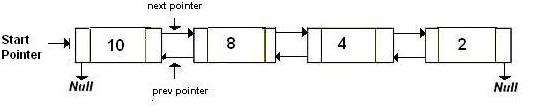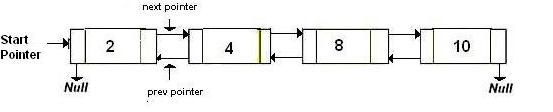GeeksforGeeks App
Open AppBrowser
Continue

# Reverse a Doubly linked list using recursion

Given a doubly linked list. Reverse it using recursion.

`Original Doubly linked list ``Reversed Doubly linked list `We have discussed
Iterative solution to reverse a Doubly Linked List

Algorithm:

1. If list is empty, return
3. If prev = NULL it means that list is fully reversed. Else reverse(head->prev)

Implementation:

## C++

 `// C++ implementation to reverse a doubly``// linked list using recursion``#include ``using` `namespace` `std;` `// a node of the doubly linked list``struct` `Node {``    ``int` `data;``    ``Node *next, *prev;``};` `// function to get a new node``Node* getNode(``int` `data)``{``    ``// allocate space``    ``Node* new_node = ``new` `Node;``    ``new_node->data = data;``    ``new_node->next = new_node->prev = NULL;``    ``return` `new_node;``}` `// function to insert a node at the beginning``// of the Doubly Linked List``void` `push(Node** head_ref, Node* new_node)``{``    ``// since we are adding at the beginning,``    ``// prev is always NULL``    ``new_node->prev = NULL;` `    ``// link the old list of the new node``    ``new_node->next = (*head_ref);` `    ``// change prev of head node to new node``    ``if` `((*head_ref) != NULL)``        ``(*head_ref)->prev = new_node;` `    ``// move the head to point to the new node``    ``(*head_ref) = new_node;``}` `// function to reverse a doubly linked list``Node* Reverse(Node* node)``{``    ``// If empty list, return``    ``if` `(!node)``        ``return` `NULL;` `    ``// Otherwise, swap the next and prev``    ``Node* temp = node->next;``    ``node->next = node->prev;``    ``node->prev = temp;` `    ``// If the prev is now NULL, the list``    ``// has been fully reversed``    ``if` `(!node->prev)``        ``return` `node;` `    ``// Otherwise, keep going``    ``return` `Reverse(node->prev);``}` `// Function to print nodes in a given doubly``// linked list``void` `printList(Node* head)``{``    ``while` `(head != NULL) {``        ``cout << head->data << ``" "``;``        ``head = head->next;``    ``}``}` `// Driver program to test above``int` `main()``{``    ``// Start with the empty list``    ``Node* head = NULL;` `    ``// Create doubly linked: 10<->8<->4<->2 */``    ``push(&head, getNode(2));``    ``push(&head, getNode(4));``    ``push(&head, getNode(8));``    ``push(&head, getNode(10));``    ``cout << ``"Original list: "``;``    ``printList(head);` `    ``// Reverse doubly linked list``    ``head = Reverse(head);``    ``cout << ``"\nReversed list: "``;``    ``printList(head);``    ``return` `0;``}`

## Java

 `// Java implementation to reverse a doubly``// linked list using recursion``class` `GFG``{``    ` `// a node of the doubly linked list``static` `class` `Node``{``    ``int` `data;``    ``Node next, prev;``};` `// function to get a new node``static` `Node getNode(``int` `data)``{``    ``// allocate space``    ``Node new_node = ``new` `Node();``    ``new_node.data = data;``    ``new_node.next = new_node.prev = ``null``;``    ``return` `new_node;``}` `// function to insert a node at the beginning``// of the Doubly Linked List``static` `Node push(Node head_ref, Node new_node)``{``    ``// since we are adding at the beginning,``    ``// prev is always null``    ``new_node.prev = ``null``;` `    ``// link the old list of the new node``    ``new_node.next = (head_ref);` `    ``// change prev of head node to new node``    ``if` `((head_ref) != ``null``)``        ``(head_ref).prev = new_node;` `    ``// move the head to point to the new node``    ``(head_ref) = new_node;``    ``return` `head_ref;``}` `// function to reverse a doubly linked list``static` `Node Reverse(Node node)``{``    ``// If empty list, return``    ``if` `(node == ``null``)``        ``return` `null``;` `    ``// Otherwise, swap the next and prev``    ``Node temp = node.next;``    ``node.next = node.prev;``    ``node.prev = temp;` `    ``// If the prev is now null, the list``    ``// has been fully reversed``    ``if` `(node.prev == ``null``)``        ``return` `node;` `    ``// Otherwise, keep going``    ``return` `Reverse(node.prev);``}` `// Function to print nodes in a given doubly``// linked list``static` `void` `printList(Node head)``{``    ``while` `(head != ``null``)``    ``{``        ``System.out.print( head.data + ``" "``);``        ``head = head.next;``    ``}``}` `// Driver code``public` `static` `void` `main(String args[])``{``    ``// Start with the empty list``    ``Node head = ``null``;` `    ``// Create doubly linked: 10<.8<.4<.2 /``    ``head = push(head, getNode(``2``));``    ``head = push(head, getNode(``4``));``    ``head = push(head, getNode(``8``));``    ``head = push(head, getNode(``10``));``    ``System.out.print( ``"Original list: "``);``    ``printList(head);` `    ``// Reverse doubly linked list``    ``head = Reverse(head);``    ``System.out.print(``"\nReversed list: "``);``    ``printList(head);``}``}` `// This code is contributed by Arnab Kundu`

## Python3

 `# Python3 implementation to reverse a doubly``# linked list using recursion``import` `math` `# a node of the doubly linked list``class` `Node:``    ``def` `__init__(``self``, data):``        ``self``.data ``=` `data``        ``self``.``next` `=` `None` `# function to get a new node``def` `getNode(data):``    ` `    ``# allocate space``    ``new_node ``=` `Node(data)``    ``new_node.data ``=` `data``    ``new_node.``next` `=` `new_node.prev ``=` `None``    ``return` `new_node` `# function to insert a node at the beginning``# of the Doubly Linked List``def` `push(head_ref, new_node):``    ` `    ``# since we are adding at the beginning,``    ``# prev is always None``    ``new_node.prev ``=` `None` `    ``# link the old list of the new node``    ``new_node.``next` `=` `head_ref` `    ``# change prev of head node to new node``    ``if` `(head_ref !``=` `None``):``        ``head_ref.prev ``=` `new_node` `    ``# move the head to point to the new node``    ``head_ref ``=` `new_node``    ``return` `head_ref` `# function to reverse a doubly linked list``def` `Reverse(node):``    ` `    ``# If empty list, return``    ``if` `not` `node:``        ``return` `None` `    ``# Otherwise, swap the next and prev``    ``temp ``=` `node.``next``    ``node.``next` `=` `node.prev``    ``node.prev ``=` `temp` `    ``# If the prev is now None, the list``    ``# has been fully reversed``    ``if` `not` `node.prev:``        ``return` `node` `    ``# Otherwise, keep going``    ``return` `Reverse(node.prev)` `# Function to print nodes in a given doubly``# linked list``def` `printList(head):``    ``while` `(head !``=` `None``) :``        ``print``(head.data, end ``=` `" "``)``        ``head ``=` `head.``next``    ` `# Driver Code``if` `__name__``=``=``'__main__'``:``    ` `    ``# Start with the empty list``    ``head ``=` `None` `    ``# Create doubly linked: 10<.8<.4<.2 */``    ``head ``=` `push(head, getNode(``2``));``    ``head ``=` `push(head, getNode(``4``));``    ``head ``=` `push(head, getNode(``8``));``    ``head ``=` `push(head, getNode(``10``));``    ``print``(``"Original list: "``, end ``=` `"")``    ``printList(head)``    ` `    ``# Reverse doubly linked list``    ``head ``=` `Reverse(head)``    ``print``(``"\nReversed list: "``, end ``=` `"")``    ``printList(head)``    ` `# This code is contributed by Srathore`

## C#

 `// C# implementation to reverse a doubly``using` `System;` `// linked list using recursion``class` `GFG``{``    ` `// a node of the doubly linked list``public` `class` `Node``{``    ``public` `int` `data;``    ``public` `Node next, prev;``};` `// function to get a new node``static` `Node getNode(``int` `data)``{``    ``// allocate space``    ``Node new_node = ``new` `Node();``    ``new_node.data = data;``    ``new_node.next = new_node.prev = ``null``;``    ``return` `new_node;``}` `// function to insert a node at the beginning``// of the Doubly Linked List``static` `Node push(Node head_ref, Node new_node)``{``    ``// since we are adding at the beginning,``    ``// prev is always null``    ``new_node.prev = ``null``;` `    ``// link the old list of the new node``    ``new_node.next = (head_ref);` `    ``// change prev of head node to new node``    ``if` `((head_ref) != ``null``)``        ``(head_ref).prev = new_node;` `    ``// move the head to point to the new node``    ``(head_ref) = new_node;``    ``return` `head_ref;``}` `// function to reverse a doubly linked list``static` `Node Reverse(Node node)``{``    ``// If empty list, return``    ``if` `(node == ``null``)``        ``return` `null``;` `    ``// Otherwise, swap the next and prev``    ``Node temp = node.next;``    ``node.next = node.prev;``    ``node.prev = temp;` `    ``// If the prev is now null, the list``    ``// has been fully reversed``    ``if` `(node.prev == ``null``)``        ``return` `node;` `    ``// Otherwise, keep going``    ``return` `Reverse(node.prev);``}` `// Function to print nodes in a given doubly``// linked list``static` `void` `printList(Node head)``{``    ``while` `(head != ``null``)``    ``{``        ``Console.Write( head.data + ``" "``);``        ``head = head.next;``    ``}``}` `// Driver code``public` `static` `void` `Main(String []argsS)``{``    ``// Start with the empty list``    ``Node head = ``null``;` `    ``// Create doubly linked: 10<.8<.4<.2 /``    ``head = push(head, getNode(2));``    ``head = push(head, getNode(4));``    ``head = push(head, getNode(8));``    ``head = push(head, getNode(10));``    ``Console.Write( ``"Original list: "``);``    ``printList(head);` `    ``// Reverse doubly linked list``    ``head = Reverse(head);``    ``Console.Write(``"\nReversed list: "``);``    ``printList(head);``}``}` `// This code is contributed by Arnab Kundu`

## Javascript

 ``

Output

```Original list: 10 8 4 2
Reversed list: 2 4 8 10 ```

Time Complexity: O(N), where N is the number of nodes in given doubly linked list.
Auxiliary Space: O(N), due to recursion call stack.

My Personal Notes arrow_drop_up